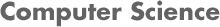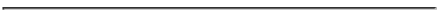### CS534 Artificial Intelligence Homework 4 - Fall 2007

#### PROF. CAROLINA RUIZ

Due Date: At the beginning of class on October 4, 2007• Read Sections 7.1-7.5, and 7.8 of the textbook.

• Problems: You need to turn in written solutions ONLY TO THE PROBLEMS MARKED WITH ONE ASTERISK "*" BELOW at the beginning of class when the homework is due. Solve the other problems before the class also so that you can ask questions about them in preparation for Exam 1.

1. Chapter 7:
• 7.4* (Remember to prove both directions of the "if and only if"),
• 7.5* (Note that the models must assign truth values each propositional letter A, B, C, and D, not just to the propositional letters appearing in the sentence. Also, note that A ⇔ B ⇔ C is shortcut for (A ⇔ B) ⇔ C, which is also equivalent to A ⇔ (B ⇔ C) ),
• 7.6*, 7.7, 7.8*, 7.9, 7.11, 7.12

2. * Determine whether the following sentence is valid, unsatisfiable, or neither. Justify your answer with either truth tables, equivalent rewrites of the sentence, or both.
` (!! q => !p) => (p => !q) `

3. * Convert the following sentence into clausal form (that is, into disjunctions of literals):
` (p and !r) => [q => (m => (!q or t))] `